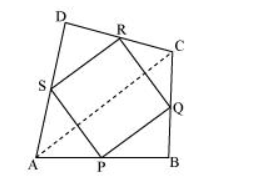# ABCD is a quadrilateral in which P, Q, R and S are mid-points of the sides AB, BC, CD and DA

Question. ABCD is a quadrilateral in which P, Q, R and S are mid-points of the sides AB, BC, CD and DA (see the given figure). AC is a diagonal. Show that:(i) $S R \| A C$ and $S R=\frac{1}{2} A C$

(ii) $P Q=S R$

(iii) $\mathrm{PQRS}$ is a parallelogram.

Solution:

(i) In ΔADC, S and R are the mid-points of sides AD and CD respectively.

In a triangle, the line segment joining the mid-points of any two sides of the triangle is parallel to the third side and is half of it.

$\therefore S R \| A C$ and $S R=\frac{1}{2} A C \ldots$(1)

(ii) In $\triangle A B C, P$ and $Q$ are mid-points of sides $A B$ and $B C$ respectively. Therefore, by using mid-point theorem,

$P Q \| A C$ and $P Q=\frac{1}{2} A C \ldots$(2)

Using equations (1) and (2), we obtain

$P Q \| S R$ and $P Q=S R$...(3)

$\Rightarrow \mathrm{PQ}=\mathrm{SR}$

(iii) From equation (3), we obtained

$\mathrm{PQ} \| \mathrm{SR}$ and $\mathrm{PQ}=\mathrm{SR}$

Clearly, one pair of opposite sides of quadrilateral $P Q R S$ is parallel and equal.

Hence, $P Q R S$ is a parallelogram.